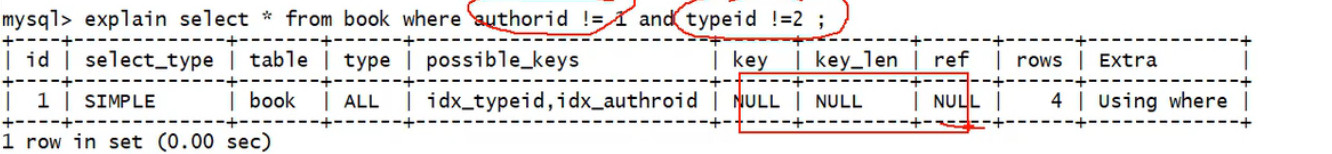# SQL优化 MySQL版 – 避免索引失效原则（一）SQL优化 MySQL版 – 避免索引失效原则（一）# 避免索引失效原则（一）

## 1.复合索引的时候，不要跨列或无序使用（最佳左前缀）

where b … and a order by c，这样很明显你顺序不对，并不满足最佳左前缀，从而导致了索引失效；

## 3.不要在索引上进行任何操作

`select * from book where authroid = 1 and typeid = 2;`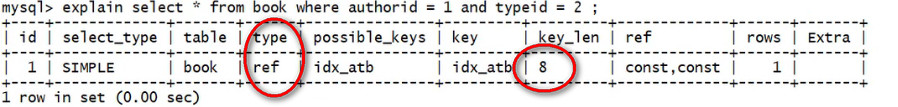`explain select * from book where authroid = 1 and typeid*2 = 2;`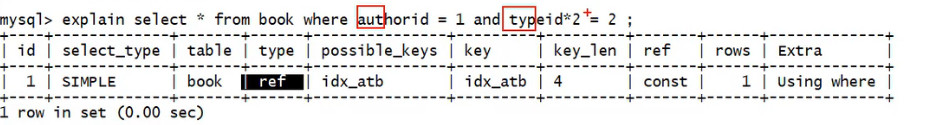`explain select * from book where authroid*2 = 1 and typeid*2 = 2;`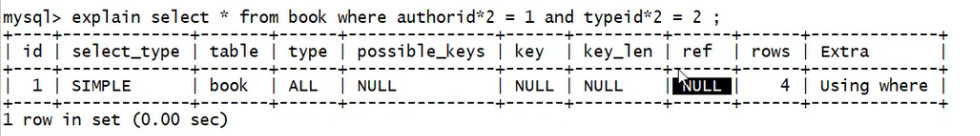## 4.对于复合索引左边失效右边全部失效

`select * from book where authroid*2 = 1 and typeid = 2;`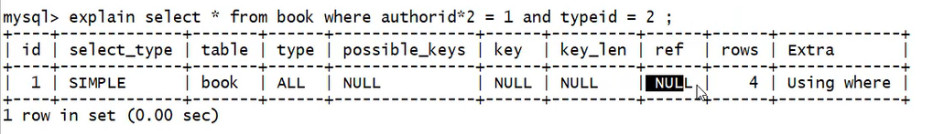## 5.复合索引不能使用不等于（！= 或 <> is null (is not null)）

`select * from book where authoid = 1 and typeid = 2`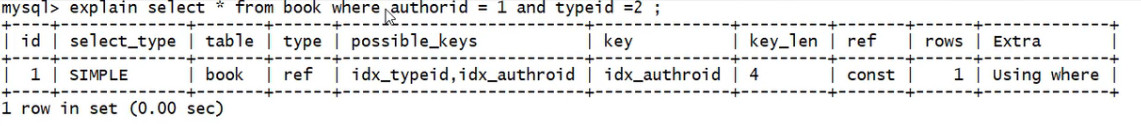`explain  select * from book where authoid ! = 1 and typeid = 2`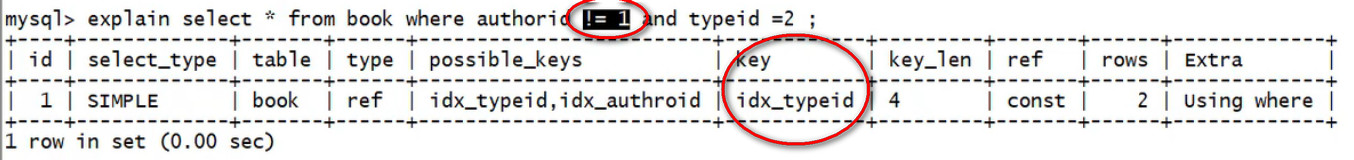`explain  select * from book where authoid ! = 1 and typeid ! = 2;`